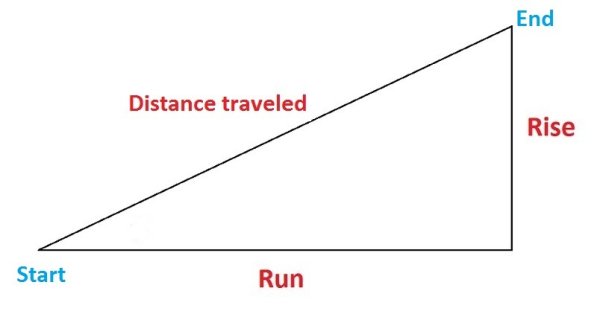08 8340 7888 info@codycorp.com.au
0
Notice: Undefined index: minicart_remove_option_text in /home/codycorp/public_html/wp-content/plugins/divi-bodycommerce/lib/features/cart-minicart/custom-cart.php on line 175

Notice: Undefined index: mini_cart_style in /home/codycorp/public_html/wp-content/plugins/divi-bodycommerce/lib/features/cart-minicart/custom-cart.php on line 176

Subtotal: \$0.00

No products in the cart.

Select Page

Grade of an object’s physical feature, a landform or a line (plane) is used to indicate the slope of the thing being measured. Applications of grade include physical features like hills, river banks and canyons and construction aspects such as roads, roof pitch and landscaping. In other words, how to find elevation grade is an important skill in many areas.

Grade is also commonly called incline or rise. Depending on the method used to finding elevation grade, it can expressed in different ways like decimal, percentage and degree.

## How to Calculate Construction Grade

Before you figure out elevation grade, elevations must be taken from the starting and ending points for comparison, as well as the starting and ending distances. Keep in mind the distance travelled is not the same as the “run” (covered in the next section). ‘Distance travelled’ is the distance traversed between the starting and ending points, not necessarily the ‘run’ distance.

Also be sure all your units are the same throughout.

As a Decimal

An easy-to-remember equation for finding change in elevation as a decimal is “rise over run,” meaning the rise (the change in vertical distance) divided by the run (the change in horizontal distance). As an example, let’s say the rise is 2 and the run is 6. So, you’d take 2 over 6 (or 2/6) to get .33 – that’s your grade as a decimal.As a Percentage

The process for measuring elevation as a percentage is the same as finding elevation change as a decimal, with one extra step. So, find the rise over run. In our example, it’s 2 over 6 (2/6) – this is .33. Then simply multiply the decimal by 100 to find the percentage. In this case, 33%. A positive percentage indicates an upward slope. A positive number indicates an upward slope, and vice versa.

In Degrees

In trigonometric terms, grade is the tangent of the angle of a surface being measured to the horizontal. The larger the number, the greater the grade (or ‘tilt’). In this case, grade is expressed in degrees. For example, the elevation grade of a street could be 6.2°, meaning the street tilts upward 6.2° from horizontal (the horizon). In other words, you’re traveling uphill.

When calculating elevation slope in degrees, it’s helpful to think of the problem as a right triangle. Using basic trigonometry, you can find grade in degrees quickly. The diagram below shows the three basic aspects you’ll need to find grade.

Simply take “rise over run” and use arctangent (tan-1) to find theta (θ) – that’s your grade in degrees. Returning to our example, the rise is 2 and the run is 6. So, you’d take 2 over 6 (or 2/6) to get .33. Take the arctangent of .33, which is 18.26. Therefore, your grade is 18.26°. A positive number indicates an upward slope, and vice versa.Below is the Calculator for determining the required Grade Percentage for your next construction project.

## Practical Application of Calculating and Finding Elevation Grade

The rise over run equation can be applied to set simple grading for patios, walkways or driveways. Every project is different and there is no single way to set grade stakes for each and every job site.

We hope you found this Construction grade Calculator useful and use it in your daily tasks on site. Keep up to date with our posts for more useful tools like this in the future.